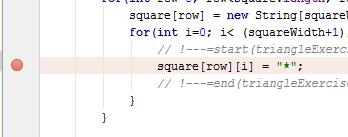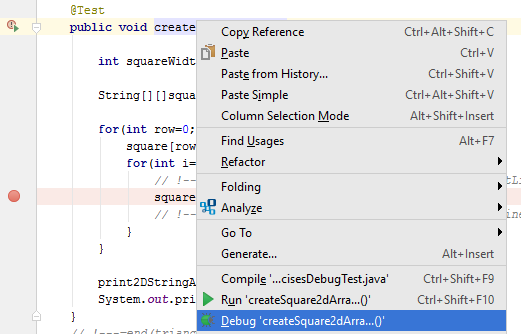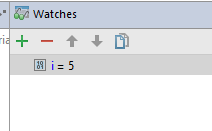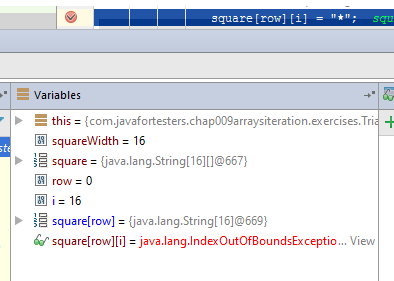4 minute read - IntelliJ Java For Testers JUnit

# How to debug Java with IntelliJ: breakpoints, evaluate expression, watches and variable view

Jul 19, 2016

I have deliberately created a failing test. Obviously deliberately since I would never ever create such simple issues in code. I’m far too experienced for that. :)

Even though these issues were created deliberately I still need to know how to debug code. In this blog post I’ll describe how I debug Java using IntelliJ.

• You can also ‘watch’ this post on YouTube

I have some code which fills a dynamic array with “*” to simulate a set of ‘pixels’ - whatever.

But it doesn’t work.

``````@Test
public void createSquare2dArray(){

int squareWidth = 16;

String[][]square = new String [squareWidth][];

for(int row=0; row<square.length; row++){
square[row] = new String[squareWidth];
for(int i=0; i< (squareWidth+1); i++){
square[row][i] = "*";
}
}

print2DStringArrayIterators(square);
System.out.println("");
}
``````

It throws an error on the line

``````    square[row][i] = "*";
``````

``````java.lang.ArrayIndexOutOfBoundsException: 16
at com.javafortesters.chap009arraysiteration.exercises.
TriangleExercisesDebugTest.
createSquare2dArray(TriangleExercisesDebugTest.java:40)
``````

It will be fairly obvious to most people what is going on but if I put a breakpoint on the line that is failing then I can Debug the @Test method and step through the code to see what is going on.Oh look, a breakpoint.Then run in debug mode.When the test is running in debug and has stopped at a breakpoint, I can:

• see all the current variables,
• expand them when they are an object instance of some sort
• resume execution (stop at next breakpoint)
• step over the line to advance execution bit by bit
• step into the code to advance execution, but into the method implementation

I can also highlight code and “Evaluate Expression”.This lets me execute code on the fly and experiment with different code constructs.

e.g. I can evaluate `square[row][i]` note that this is not the full line, just ‘code’.

I can run that and experiment with it

e.g.

• `square[row][i]` returns `null` because we haven’t set the value yet
• `square[row]` also returns `null` because we haven’t set the value yet
• `square[row]` reports `java.lang.IndexOutOfBoundsException: Invalid array range: 16 to 16`

That `IndexOutOfBoundsException` seems suspiciously close to the error I saw when I ran the test.

I could add a ‘Watch’ for `i` even though it is obvious in the Variables list by clicking the green `+` symbol in the ‘Watch’ section.So if I step through until `i==16` then I can also see in the variables list the exception will be thrown - even though the line hasn’t executed yet.And we know the problem is that I copied the code from a previous ’triangle exercise’

``````String[][]triangle = new String [];

for(int row=0; row<triangle.length; row++){
triangle[row] = new String[row+1];
for(int i=0; i< (row+1); i++){
triangle[row][i] = "*";
}
}
``````

And accidentally left a `+1` in the loop condition.

I fix that.

``````for(int row=0; row<square.length; row++){
square[row] = new String[squareWidth];
for(int i=0; i< squareWidth; i++){
square[row][i] = "*";
}
}
``````

And then I have a problem in my next method.

Instead of printing out a `16x16` square of `*`

It prints out:

``````****************
``````

And here is the horror in all its glory:

``````public void print2DStringArrayIterators(String [][]multi){

Iterable<String[]> outerList = Arrays.asList(multi);
Iterator<String[]> outer = outerList.iterator();

while(outer.hasNext()){
String[] innerMulti = outer.next();
Iterable<String> innerList = Arrays.asList(innerMulti);
Iterator<String> inner = innerList.iterator();

while(inner.hasNext()){
String pixel = inner.next();
System.out.print(pixel);
}
System.out.println("");
break;
}
}
``````

I decided to “get fancy” and use iterators and lists instead of just `for` loops with arrays.
But I didn’t get fancy enough. I should have wrapped my `print2DStringArrayIterators` with `@Test` methods and made sure it worked before I used it.

• Me: “But it prints out, I couldn’t unit test it”
• Other Me: “You could have written a method that returned the output, and we could have used `@Test` to assert on its functionality
• Me: …
• Other Me: Yeah, exactly.

But I didn’t do that. So I have to debug it instead.

If I breakpoint the line where the method is called:

``````print2DStringArrayIterators(square);
``````

Then I can “step into” the method and debug from there.

And As I step through the method, the problem is obvious - the `break;` that I added by mistake.

## Summary

OK. So these were pretty poor examples, but, they are also very similar to code examples that I’ve received emails from, and probably wrote myself back in the day (Monday, or yesterday, or something).
Basics:

• Yes we prefer to TDD our code
• if we don’t TDD we better learn how to debug
• use breakpoints
• step through code
• use the ‘variables view’
• setup up ‘Watches’ if you have a lot of variables
• use ‘Evaluate Expression’ to experiment in the running code
• use Resume to run to next breakpoint
• use Step Into to move to the next level of code in a method

Try it and see.